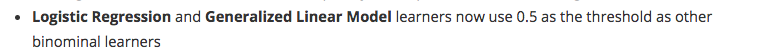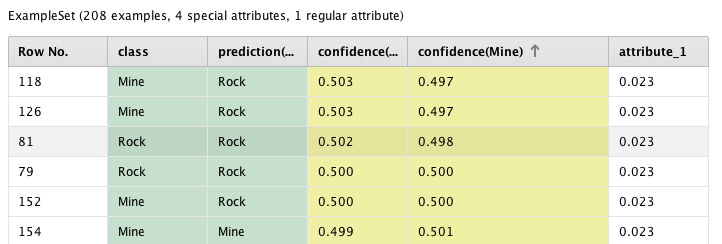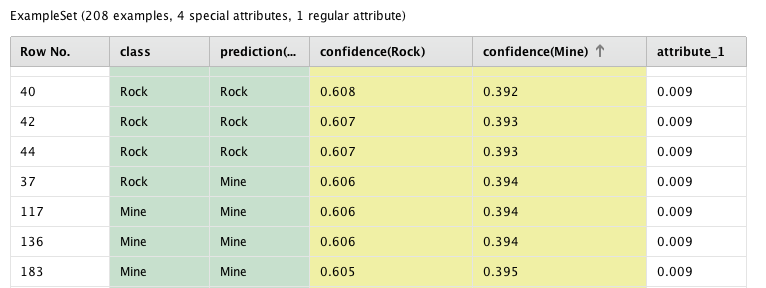# "Threshold for Logistic Regression (Inconsistent behavior)"

Member Posts: 270Unicorn
edited June 2019 in HelpFor the sake of consistency with other classifiers, I was glad to see this "bug" fixed. However, there is still some weird behavior if the model comes out of a X-validation operator. Take a look at the code below:

- When I use the operator directly I get the expected behavior (Observation is classified as Mine when confidence(Mine)>0.5)- But when I use it coming out of a X-validation operator something else happens (Observation is classified as Mine when confidence(Mine)>0.383)Tagged:

• Member Posts: 270Unicorn
`<?xml version="1.0" encoding="UTF-8"?><process version="7.6.001">  <context>    <input/>    <output/>    <macros/>  </context>  <operator activated="true" class="process" compatibility="6.0.002" expanded="true" name="Process">    <process expanded="true">      <operator activated="true" class="retrieve" compatibility="7.6.001" expanded="true" height="68" name="Retrieve Sonar" width="90" x="45" y="136">        <parameter key="repository_entry" value="//Samples/data/Sonar"/>      </operator>      <operator activated="true" class="select_attributes" compatibility="7.6.001" expanded="true" height="82" name="Select Attributes" width="90" x="179" y="136">        <parameter key="attribute_filter_type" value="single"/>        <parameter key="attribute" value="attribute_1"/>      </operator>      <operator activated="true" class="multiply" compatibility="7.6.001" expanded="true" height="103" name="Multiply" width="90" x="313" y="136"/>      <operator activated="true" class="h2o:logistic_regression" compatibility="7.6.001" expanded="true" height="124" name="Logistic Regression (2)" width="90" x="447" y="340"/>      <operator activated="true" class="apply_model" compatibility="7.6.001" expanded="true" height="82" name="Apply Model (3)" width="90" x="618" y="289">        <list key="application_parameters"/>      </operator>      <operator activated="true" class="concurrency:cross_validation" compatibility="7.6.001" expanded="true" height="145" name="Cross Validation" width="90" x="447" y="34">        <parameter key="use_local_random_seed" value="true"/>        <process expanded="true">          <operator activated="true" class="h2o:logistic_regression" compatibility="7.5.000" expanded="true" height="124" name="Logistic Regression" width="90" x="179" y="34"/>          <connect from_port="training set" to_op="Logistic Regression" to_port="training set"/>          <connect from_op="Logistic Regression" from_port="model" to_port="model"/>          <portSpacing port="source_training set" spacing="0"/>          <portSpacing port="sink_model" spacing="0"/>          <portSpacing port="sink_through 1" spacing="0"/>        </process>        <process expanded="true">          <operator activated="true" class="apply_model" compatibility="7.1.001" expanded="true" height="82" name="Apply Model" width="90" x="112" y="34">            <list key="application_parameters"/>          </operator>          <operator activated="true" class="performance_binominal_classification" compatibility="7.6.001" expanded="true" height="82" name="Performance" width="90" x="246" y="30">            <parameter key="kappa" value="true"/>            <parameter key="AUC" value="true"/>          </operator>          <connect from_port="model" to_op="Apply Model" to_port="model"/>          <connect from_port="test set" to_op="Apply Model" to_port="unlabelled data"/>          <connect from_op="Apply Model" from_port="labelled data" to_op="Performance" to_port="labelled data"/>          <connect from_op="Performance" from_port="performance" to_port="performance 1"/>          <connect from_op="Performance" from_port="example set" to_port="test set results"/>          <portSpacing port="source_model" spacing="0"/>          <portSpacing port="source_test set" spacing="0"/>          <portSpacing port="source_through 1" spacing="0"/>          <portSpacing port="sink_test set results" spacing="0"/>          <portSpacing port="sink_performance 1" spacing="0"/>          <portSpacing port="sink_performance 2" spacing="0"/>        </process>      </operator>      <operator activated="true" class="apply_model" compatibility="7.6.001" expanded="true" height="82" name="Apply Model (2)" width="90" x="581" y="34">        <list key="application_parameters"/>      </operator>      <connect from_op="Retrieve Sonar" from_port="output" to_op="Select Attributes" to_port="example set input"/>      <connect from_op="Select Attributes" from_port="example set output" to_op="Multiply" to_port="input"/>      <connect from_op="Multiply" from_port="output 1" to_op="Cross Validation" to_port="example set"/>      <connect from_op="Multiply" from_port="output 2" to_op="Logistic Regression (2)" to_port="training set"/>      <connect from_op="Logistic Regression (2)" from_port="model" to_op="Apply Model (3)" to_port="model"/>      <connect from_op="Logistic Regression (2)" from_port="exampleSet" to_op="Apply Model (3)" to_port="unlabelled data"/>      <connect from_op="Apply Model (3)" from_port="labelled data" to_port="result 2"/>      <connect from_op="Cross Validation" from_port="model" to_op="Apply Model (2)" to_port="model"/>      <connect from_op="Cross Validation" from_port="example set" to_op="Apply Model (2)" to_port="unlabelled data"/>      <connect from_op="Apply Model (2)" from_port="labelled data" to_port="result 1"/>      <portSpacing port="source_input 1" spacing="0"/>      <portSpacing port="sink_result 1" spacing="0"/>      <portSpacing port="sink_result 2" spacing="0"/>      <portSpacing port="sink_result 3" spacing="0"/>    </process>  </operator></process>`